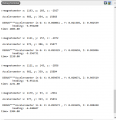# LSM303C how to compute compass heading using accelerometer and magnetometer data

#### zazas321

Joined Nov 29, 2015
926
I am using LSM303C 3D compass module to measure accelerometer and magnetometer. Ideally I would like to know a compass heading which would tell me which direction I am facing, however, I am still not unsure how would you do that. I have tried using FusionCompass library which takes the cross product of my accelerometer and magnetometer data, but the results are not quite as expected.

My accelerometer data seems fine - when the device is flat on the surface, I am reading(converted to G):

X=0g
Y=0g
Z=1g

Is there any way to confirm whether the magnetometer data is correct?

My debug window:C:
void magxl_test() {
if(magxl_init() < 0) {
#ifdef DEBUG_PRINT
#endif
}
else {
#ifdef DEBUG_PRINT
debug_printf("MAG not responding\n");
#endif
}
while(1);
}
uint8_t reg = WHO_AM_I_A;

FusionVector3 accelerometer = {
.axis.x = 0.0f,
.axis.y = 0.0f,
.axis.z = 0.0f,
};

FusionVector3 magnetometer = {
.axis.x = 0.0f,
.axis.y = 0.0f,
.axis.z = 0.0f,
};

xyzData_t magdata; //create a variable named magdata which contains structure x y and z
xyzData_t xldata;
int dataCount;
float curent_time=HAL_GetTick();

while(1) {

magxl_mag_getData(&magdata);
dataCount = magxl_xl_getFIFOData(xldata);
// Calculate offset for each axis and substract from reading
float offset_X= (X_MAX + X_MIN)/2;
float offset_Y= (Y_MAX + Y_MIN)/2;
float offset_Z= (Z_MAX + Z_MIN)/2;
#ifdef DEBUG_PRINT
debug_printf("\n\n>>magnetometer x: %i, y: %i, z: %i\n", magdata.x, magdata.y, magdata.z);

debug_printf("\n Accelerometer x: %i, y: %i, z: %i\n", xldata->x, xldata->y, xldata->z);
#endif
//Convert to G value
//Z-Axis
//Z@1G = 15500
//Z@-1G = -16800
//Z Centre Point = (15500 - 16800) / 2 = -650
//Z Range = 15500 + 16800 = 32300
//G Per Sample Z = 2.0G /32300 = 0.00006191950464f

// 2G divided by full scale for each axis
GPerSample.z = 2 / (Z_MAX - Z_MIN);
GPerSample.y = 2 / (Y_MAX - Y_MIN);
GPerSample.x = 2 / (X_MAX - X_MIN);
// Calculate offset for each axis and substract from reading

float zG = (GPerSample.z *  (xldata->z));
float xG = (GPerSample.x *  (xldata->x));
float yG = (GPerSample.y *  (xldata->y));
#ifdef DEBUG_PRINT
debug_printf("\n DEBUG****Accelerometer in G: X: %f , Y: %f, Z: %f\n", xG, yG, zG);
#endif

magnetometer.axis.x = ((float)magdata.x);
magnetometer.axis.y = ((float)magdata.y);
magnetometer.axis.z = ((float)magdata.z);
accelerometer.axis.x = ((float) xldata->x);
accelerometer.axis.y = ((float) xldata->y);
accelerometer.axis.z = ((float) xldata->z);

#ifdef DEBUG_PRINT
// debug_printf("\n\n>>magnetometer x: %i, y: %i, z: %i\n", magdata.x, magdata.y, magdata.z);
#endif

}